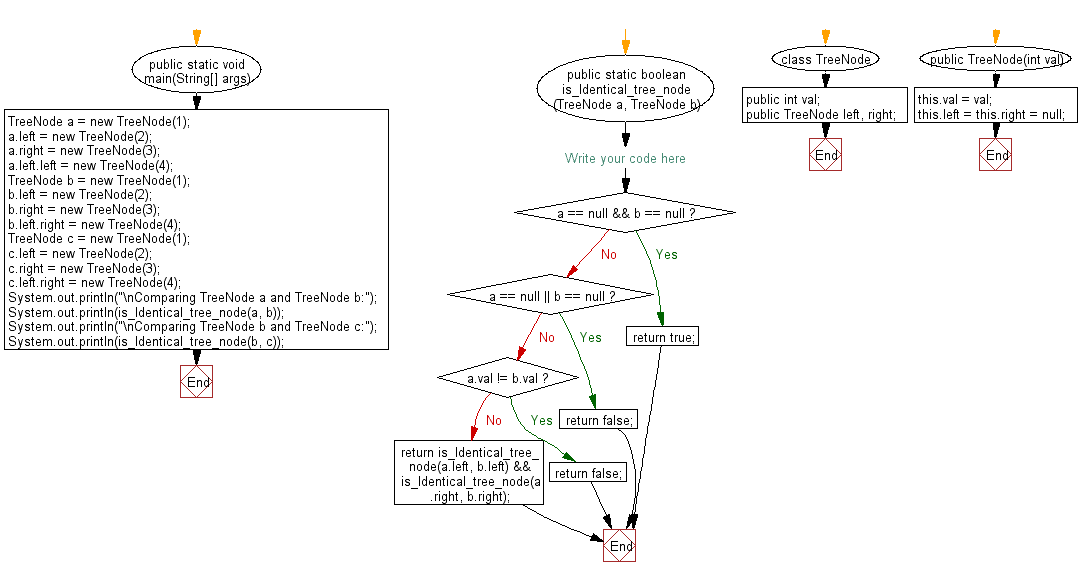﻿ Java programming exercises: Check if two binary trees are identical or not - w3resource# Java Exercises: Check if two binary trees are identical or not

## Java Basic: Exercise-182 with Solution

Write a Java program to check if two binary trees are identical or not. Assume that two binary trees have the same structure and every identical position has the same value.

Sample Solution:

Java Code:

``````import java.util.*;
public class Solution {
public static void main(String[] args) {
TreeNode a = new TreeNode(1);
a.left = new TreeNode(2);
a.right = new TreeNode(3);
a.left.left = new TreeNode(4);

TreeNode b = new TreeNode(1);
b.left = new TreeNode(2);
b.right = new TreeNode(3);
b.left.right = new TreeNode(4);

TreeNode c = new TreeNode(1);
c.left = new TreeNode(2);
c.right = new TreeNode(3);
c.left.right = new TreeNode(4);

System.out.println("\nComparing TreeNode a and TreeNode b:");
System.out.println(is_Identical_tree_node(a, b));
System.out.println("\nComparing TreeNode b and TreeNode c:");
System.out.println(is_Identical_tree_node(b, c));
}

public static boolean is_Identical_tree_node(TreeNode a, TreeNode b) {
if (a == null && b == null) return true;
if (a == null || b == null) {
return false;
}
if (a.val != b.val) return false;
return is_Identical_tree_node(a.left, b.left) &&
is_Identical_tree_node(a.right, b.right);
}
}
class TreeNode {
public int val;
public TreeNode left, right;
public TreeNode(int val) {
this.val = val;
this.left = this.right = null;
}
}
```
```

Sample Output:

```Comparing TreeNode a and TreeNode b:
false

Comparing TreeNode b and TreeNode c:
true
```

Flowchart:Java Code Editor:

Company:

What is the difficulty level of this exercise?

﻿

## Java: Tips of the Day

Array vs ArrayLists:

The main difference between these two is that an Array is of fixed size so once you have created an Array you cannot change it but the ArrayList is not of fixed size. You can create instances of ArrayLists without specifying its size. So if you create such instances of an ArrayList without specifying its size Java will create an instance of an ArrayList of default size.

Once an ArrayList is full it re-sizes itself. In fact, an ArrayList is internally supported by an array. So when an ArrayList is resized it will slow down its performance a bit as the contents of the old Array must be copied to a new Array.

At the same time, it's compulsory to specify the size of an Array directly or indirectly while creating it. And also Arrays can store both primitives and objects while ArrayLists only can store objects.

Ref: https://bit.ly/3o8L2KH